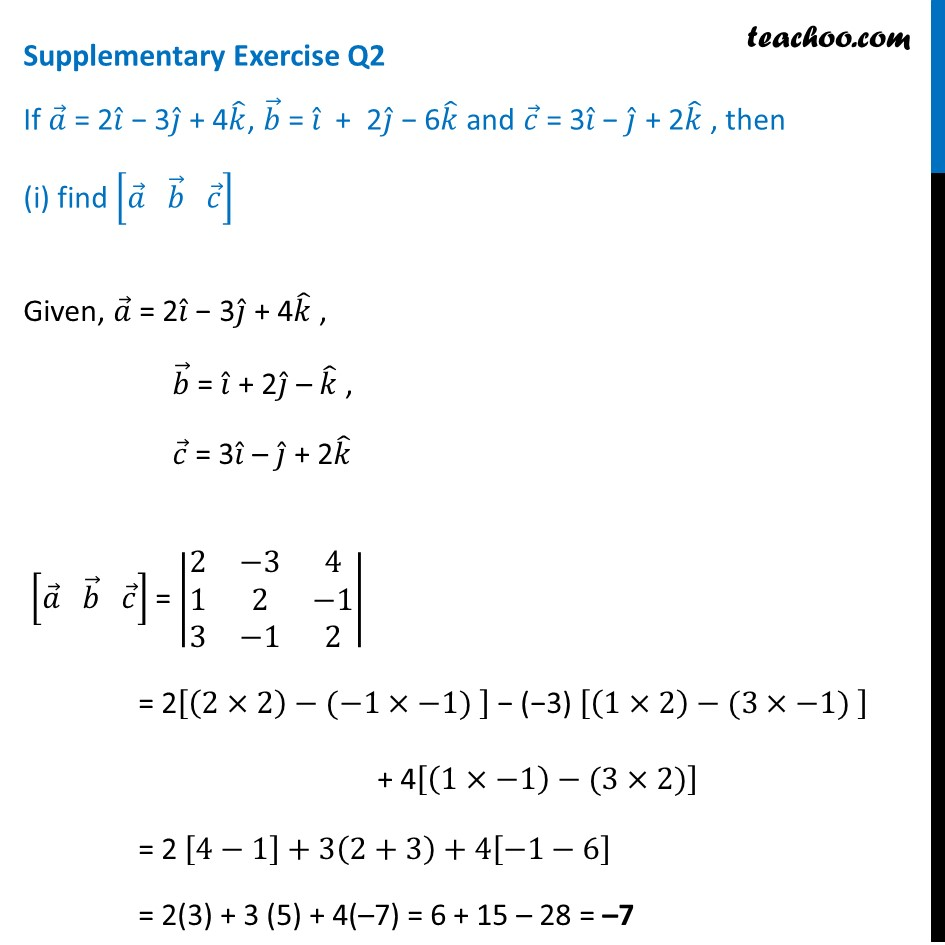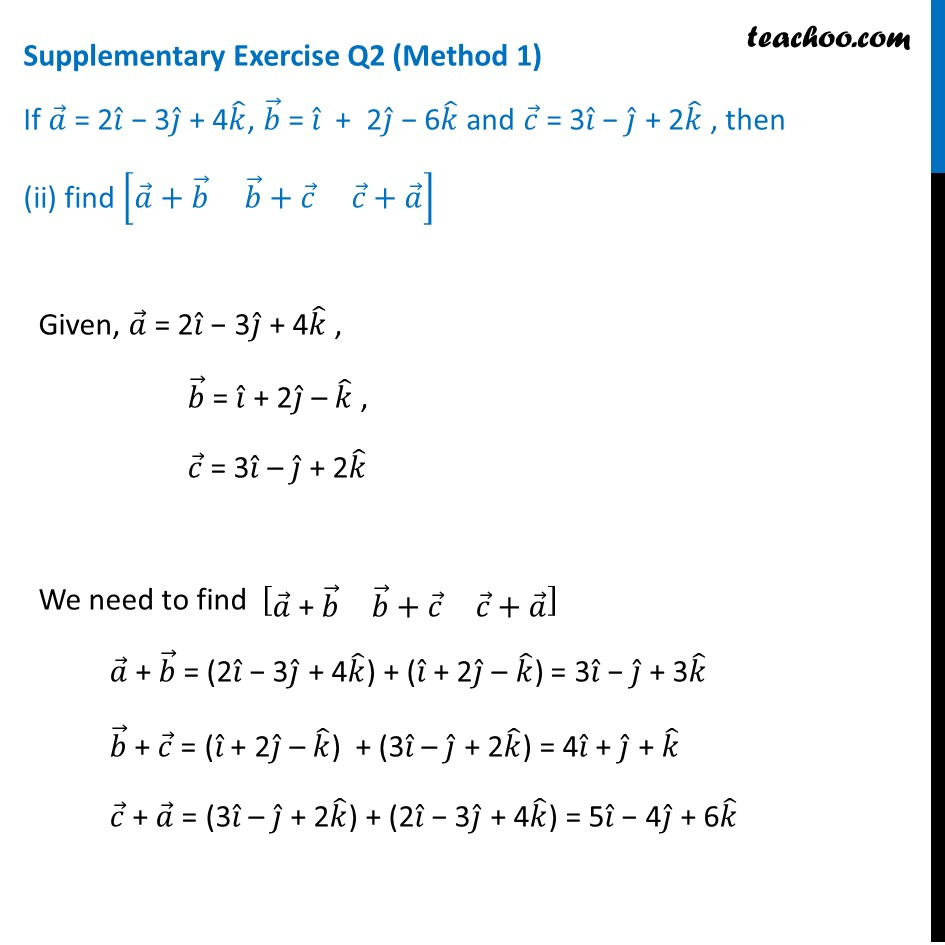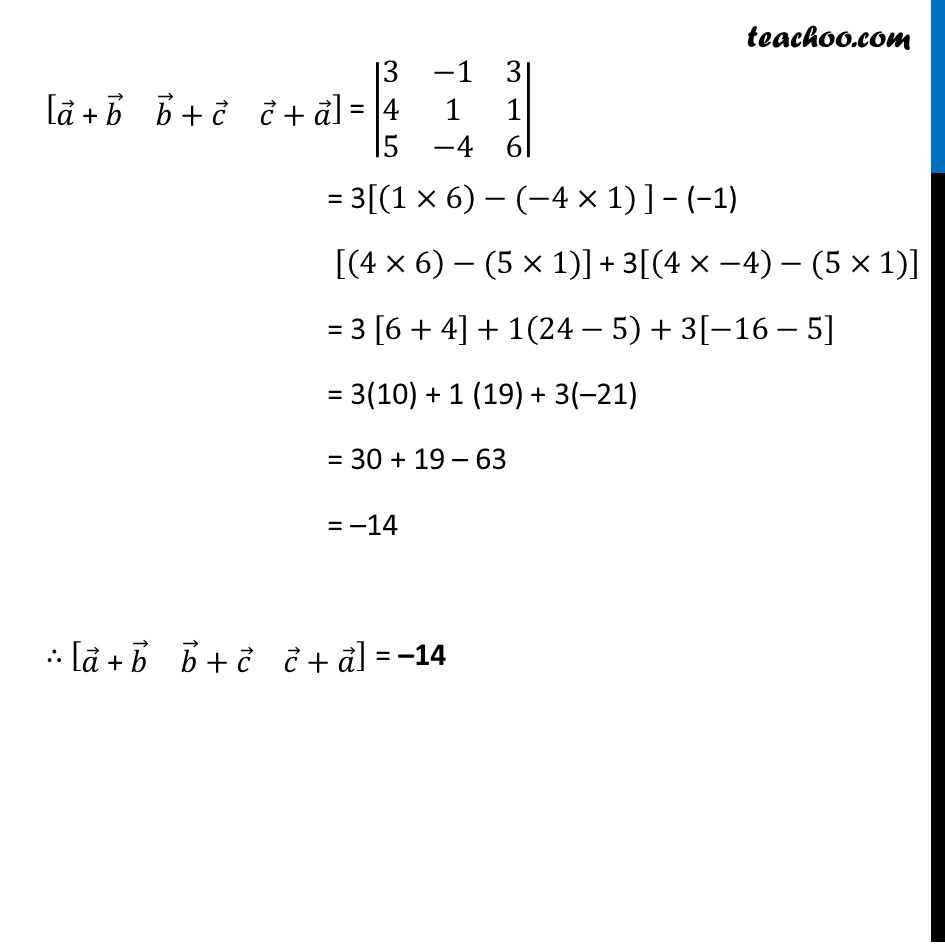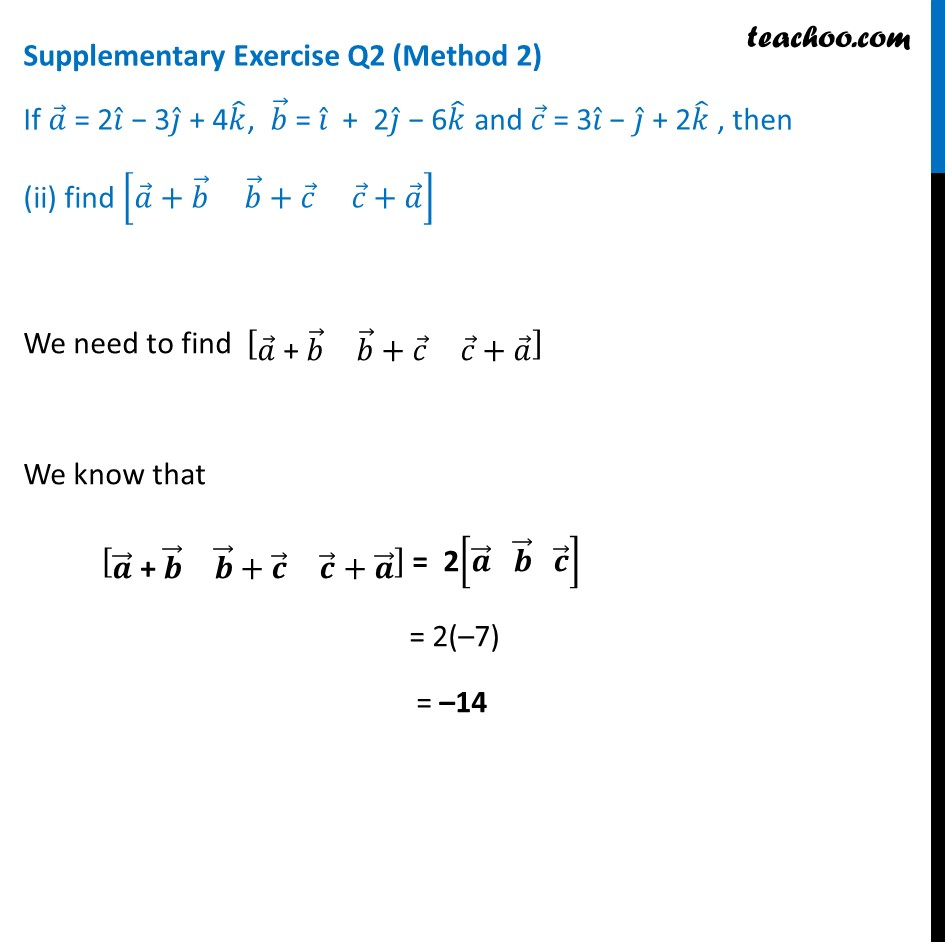Supplementary examples and questions from CBSE

Chapter 10 Class 12 Vector Algebra
Serial order wiseLearn in your speed, with individual attention - Teachoo Maths 1-on-1 Class

### Transcript

Supplementary Exercise Q2 If 𝑎 ⃗ = 2𝑖 ̂ − 3𝑗 ̂ + 4𝑘 ̂, 𝑏 ⃗ = 𝑖 ̂ + 2𝑗 ̂ − 6𝑘 ̂ and 𝑐 ⃗ = 3𝑖 ̂ − 𝑗 ̂ + 2𝑘 ̂ , then (i) find [𝑎 ⃗ 𝑏 ⃗ 𝑐 ⃗ ] Given, 𝑎 ⃗ = 2𝑖 ̂ − 3𝑗 ̂ + 4𝑘 ̂ , 𝑏 ⃗ = 𝑖 ̂ + 2𝑗 ̂ – 𝑘 ̂ , 𝑐 ⃗ = 3𝑖 ̂ – 𝑗 ̂ + 2𝑘 ̂ [𝑎 ⃗" " 𝑏 ⃗" " 𝑐 ⃗ ] = |■8(2&−3&4@1&2&−1@3&−1&2)| = 2[(2×2)−(−1×−1) ] − (−3) [(1×2)−(3×−1) ] + 4[(1×−1)−(3×2)] = 2 [4−1]+3(2+3)+4[−1−6] = 2(3) + 3 (5) + 4(–7) = 6 + 15 – 28 = –7 Supplementary Exercise Q2 (Method 1) If 𝑎 ⃗ = 2𝑖 ̂ − 3𝑗 ̂ + 4𝑘 ̂, 𝑏 ⃗ = 𝑖 ̂ + 2𝑗 ̂ − 6𝑘 ̂ and 𝑐 ⃗ = 3𝑖 ̂ − 𝑗 ̂ + 2𝑘 ̂ , then (ii) find [𝑎 ⃗+𝑏 ⃗ 𝑏 ⃗+𝑐 ⃗ 𝑐 ⃗+𝑎 ⃗ ] Given, 𝑎 ⃗ = 2𝑖 ̂ − 3𝑗 ̂ + 4𝑘 ̂ , 𝑏 ⃗ = 𝑖 ̂ + 2𝑗 ̂ – 𝑘 ̂ , 𝑐 ⃗ = 3𝑖 ̂ – 𝑗 ̂ + 2𝑘 ̂ We need to find [■8(𝑎 ⃗" + " 𝑏 ⃗&𝑏 ⃗+𝑐 ⃗&𝑐 ⃗+𝑎 ⃗ )] 𝑎 ⃗" + " 𝑏 ⃗ = (2𝑖 ̂ − 3𝑗 ̂ + 4𝑘 ̂) + (𝑖 ̂ + 2𝑗 ̂ – 𝑘 ̂) = 3𝑖 ̂ − 𝑗 ̂ + 3𝑘 ̂ 𝑏 ⃗ + 𝑐 ⃗ = (𝑖 ̂ + 2𝑗 ̂ – 𝑘 ̂) + (3𝑖 ̂ – 𝑗 ̂ + 2𝑘 ̂) = 4𝑖 ̂ + 𝑗 ̂ + 𝑘 ̂ 𝑐 ⃗ + 𝑎 ⃗ = (3𝑖 ̂ – 𝑗 ̂ + 2𝑘 ̂) + (2𝑖 ̂ − 3𝑗 ̂ + 4𝑘 ̂) = 5𝑖 ̂ − 4𝑗 ̂ + 6𝑘 ̂ [■8(𝑎 ⃗" + " 𝑏 ⃗&𝑏 ⃗+𝑐 ⃗&𝑐 ⃗+𝑎 ⃗ )] = |■8(3&−1&3@4&1&1@5&−4&6)| = 3[(1×6)−(−4×1) ] − (−1) [(4×6)−(5×1)] + 3[(4×−4)−(5×1)] = 3 [6+4]+1(24−5)+3[−16−5] = 3(10) + 1 (19) + 3(–21) = 30 + 19 – 63 = –14 ∴ [■8(𝑎 ⃗" + " 𝑏 ⃗&𝑏 ⃗+𝑐 ⃗&𝑐 ⃗+𝑎 ⃗ )] = –14 Supplementary Exercise Q2 (Method 2) If 𝑎 ⃗ = 2𝑖 ̂ − 3𝑗 ̂ + 4𝑘 ̂, 𝑏 ⃗ = 𝑖 ̂ + 2𝑗 ̂ − 6𝑘 ̂ and 𝑐 ⃗ = 3𝑖 ̂ − 𝑗 ̂ + 2𝑘 ̂ , then (ii) find [𝑎 ⃗+𝑏 ⃗ 𝑏 ⃗+𝑐 ⃗ 𝑐 ⃗+𝑎 ⃗ ] We need to find [■8(𝑎 ⃗" + " 𝑏 ⃗&𝑏 ⃗+𝑐 ⃗&𝑐 ⃗+𝑎 ⃗ )] We know that [■8(𝒂 ⃗" + " 𝒃 ⃗&𝒃 ⃗+𝒄 ⃗&𝒄 ⃗+𝒂 ⃗ )] = 2[𝒂 ⃗" " 𝒃 ⃗" " 𝒄 ⃗ ] = 2(–7) = –14